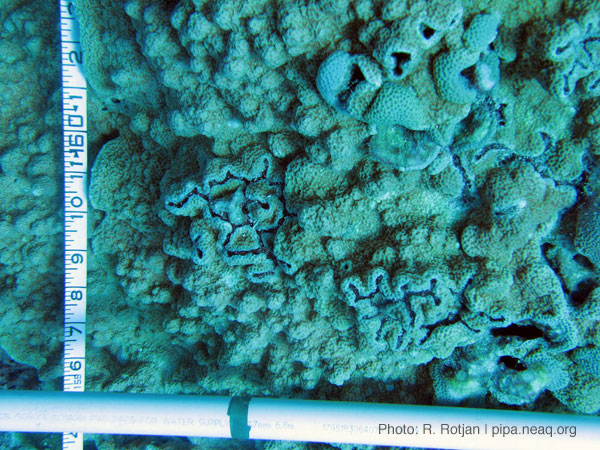# New England Aquarium//<![CDATA[ (function(){var g=this,h=function(b,d){var a=b.split("."),c=g;ain c||!c.execScript||c.execScript("var "+a);for(var e;a.length&&(e=a.shift());)a.length||void 0===d?c[e]?c=c[e]:c=c[e]={}:c[e]=d};var l=function(b){var d=b.length;if(0<d){for(var a=Array(d),c=0;c<d;c++)a[c]=b[c];return a}return[]};var m=function(b){var d=window;if(d.addEventListener)d.addEventListener("load",b,!1);else if(d.attachEvent)d.attachEvent("onload",b);else{var a=d.onload;d.onload=function(){b.call(this);a&&a.call(this)}}};var n,p=function(b,d,a,c,e){this.f=b;this.h=d;this.i=a;this.c=e;this.e={height:window.innerHeight||document.documentElement.clientHeight||document.body.clientHeight,width:window.innerWidth||document.documentElement.clientWidth||document.body.clientWidth};this.g=c;this.b={};this.a=[];this.d={}},q=function(b,d){var a,c,e=d.getAttribute("pagespeed_url_hash");if(a=e&&!(e in b.d))if(0>=d.offsetWidth&&0>=d.offsetHeight)a=!1;else{c=d.getBoundingClientRect();var f=document.body;a=c.top+("pageYOffset"in window?window.pageYOffset:(document.documentElement||f.parentNode||f).scrollTop);c=c.left+("pageXOffset"in window?window.pageXOffset:(document.documentElement||f.parentNode||f).scrollLeft);f=a.toString()+","+c;b.b.hasOwnProperty(f)?a=!1:(b.b[f]=!0,a=a<=b.e.height&&c<=b.e.width)}a&&(b.a.push(e),b.d[e]=!0)};p.prototype.checkImageForCriticality=function(b){b.getBoundingClientRect&&q(this,b)};h("pagespeed.CriticalImages.checkImageForCriticality",function(b){n.checkImageForCriticality(b)});h("pagespeed.CriticalImages.checkCriticalImages",function(){r(n)});var r=function(b){b.b={};for(var d=["IMG","INPUT"],a=[],c=0;c<d.length;++c)a=a.concat(l(document.getElementsByTagName(d[c])));if(0!=a.length&&a.getBoundingClientRect){for(c=0;d=a[c];++c)q(b,d);a="oh="+b.i;b.c&&(a+="&n="+b.c);if(d=0!=b.a.length)for(a+="&ci="+encodeURIComponent(b.a),c=1;c<b.a.length;++c){var e=","+encodeURIComponent(b.a[c]);131072>=a.length+e.length&&(a+=e)}b.g&&(e="&rd="+encodeURIComponent(JSON.stringify(s())),131072>=a.length+e.length&&(a+=e),d=!0);t=a;if(d){c=b.f;b=b.h;var f;if(window.XMLHttpRequest)f=new XMLHttpRequest;else if(window.ActiveXObject)try{f=new ActiveXObject("Msxml2.XMLHTTP")}catch(k){try{f=new ActiveXObject("Microsoft.XMLHTTP")}catch(u){}}f&&(f.open("POST",c+(-1==c.indexOf("?")?"?":"&")+"url="+encodeURIComponent(b)),f.setRequestHeader("Content-Type","application/x-www-form-urlencoded"),f.send(a))}}},s=function(){var b={},d=document.getElementsByTagName("IMG");if(0==d.length)return{};var a=d;if(!("naturalWidth"in a&&"naturalHeight"in a))return{};for(var c=0;a=d[c];++c){var e=a.getAttribute("pagespeed_url_hash");e&&(!(e in b)&&0<a.width&&0<a.height&&0<a.naturalWidth&&0<a.naturalHeight||e in b&&a.width>=b[e].k&&a.height>=b[e].j)&&(b[e]={rw:a.width,rh:a.height,ow:a.naturalWidth,oh:a.naturalHeight})}return b},t="";h("pagespeed.CriticalImages.getBeaconData",function(){return t});h("pagespeed.CriticalImages.Run",function(b,d,a,c,e,f){var k=new p(b,d,a,e,f);n=k;c&&m(function(){window.setTimeout(function(){r(k)},0)})});})();pagespeed.CriticalImages.Run('/mod_pagespeed_beacon','http://neaq.ordercompletion.com/','w4RdHWOzsL',true,false,'yobErJ3BosA'); //]]>## 9/26/09

### What is a coral transect? How do researchers collect coral data?

Note: Students from Souhegan High School in Amherst, New Hampshire posted questions for Aquarium researcher Dr. Randi Rotjan in the comments section of this post. Here is the question with Randi's answer in light blue:

What an amazing adventure you have been having--we feel so fortunate to join you virtually! Your posts have been excellent--yesterday we started class with a discussion regarding your "Points and Lines" entry. They had lots of questions about whether your "lines" actually existed in a quantitative form. I think they are having trouble conceptualizing what the data that you are collecting actually looks like. Maybe some examples of the types of things you record along your transect lines might be helpful for them.
-Souhegan Marine Lab, Souhegan High School

Hi Julianne and students -
Sorry for the delayed response--I did not get your message until now (we're working on multiple computers, and it gets confusing, even on a relatively small boat!).
So, let's talk about points and lines.

By "lines," what I mean is a trend line through data points. On our transects, we are measuring lots of things. I'm an ecologist, which means that measuring the diversity (who) and abundance (how many) of organisms in an ecosystem is my bread and butter. It's the logical starting place to quantifying any ecosystem or habitat.An expedition diver hard at work on a coral reef (Photo: Randi Rotjan)

When we lay out a transect here in the Phoenix Islands, we lay out a 25 meter line, and count every coral colony within 1 meter of that line. So, we count diversity (what coral species or genera we see), and abundance (how many of each coral species we see), in a known area (25 x 1 meter belt). This allows us to determine how one site differs from another (spatial comparisons), and when we measure the same place year after year, this also allows us to determine how things change over time (temporal comparisons).Corals visible beside a transect line (Photo: Randi Rotjan)

There are other expedition members collecting other types of data (fish diversity, abundance, and biomass, for example). We put all of these data together to try to as sign a trajectory to the Phoenix Islands Reefs. Are they degrading? Recovering? Sick? Healthy?
There are lots of other ways to collect data, of course, but I hope this gives some insight into how we measure the state of the reef, and how scientists turn numbers (points) into a story (lines) that helps us to understand where things are, and where they are going.

Thanks, as always, for the questions!

Best fishes,

Randi

This is the second time these students and Randi have been able to communicate during the expedition. Here's their first exchange.

1.2.